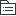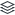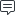# 时空大数据平台

11
1

11
|
1

## 相关模板推荐

•## 国土空间基础信息平台 —— 作品大纲

•建设原则
• 整合优化已有基础
• 设备
• 软件
• 数据
• 明确国家和行业标准
• 接口规范
• 部门对接
• 统一管理
• 按需建设
• 针对急需的业务应用
• 选择核心的数据资源
• 安全规范
• 数据开放共享机制
• 数据安全保密
•总体方向
• 国土空间工作底图
• 覆盖区域
• 涉及地上地下
• 及时更新
• 基础地理
• 土地资源
• 矿产资源
• 基础地质
• 地质环境
• 地质灾害
• 自然资源产权
•数据支撑和服务
• 国土空间工作底板
• 专业规划
• 项目实施
• 日常监管
• 分析决策
•信息化工作平台
• 国土空间工作底线
• 城镇保护区
• 生态保护区
• 农业保护区
• 基本农田保护红线
• 生态保护红线
• 城市开发边界
•管控机制
•未来应用
• 国土资源规划
• 空间现状数据
• 空间规划数据
•叠加分析
• 是否达到规划目标
• 总结原因
• 后期规划意见
• 辅助规划修编
• 规划监测
• 国土空间数据使用
• 查询平台数据资源体系
• 申请许可下载使用
• 数据脱密脱敏分级
• 国土调查
• 各类土地利用状况
• 不动产权籍调查
• 土地
• 林地
• 草原
• 海域
• 专项调查
• 基本农田
• 批而未用
• 自然资源状况
• 耕地质量评定
• 其他行业
• 国土
• 环保
• 交通
• 水利
• 农业
• 林业
•数据资源
• 大数据分析
• 数据挖掘
• 数据检索
• 数据可视化
•技术
• 分布式
• 云计算
• 大数据
• 网络通信
• 二三维一体化
• 北斗
• GIS
• RS
•难度
• 建设框架不一
• 基础平台不一
• 数据库标准不一
• 管理维护困难
•未来工程向
• 自然资源要素综合观测网络工程
• 不同时期自然资源及环境要素的变化状况及其动因
• 不同自然资源的功能特征和作业机理，形成管控机制
• 自然资源要素基础立体观测网络
• 智能化观测装备和真实性观测技术
• 自然资源全要素调查监测工程
• 建立山水林田湖草不同自然资源类型的数量和质量关键指标体系
• 建立山水林田湖草自然资源全要素统一的调查标准
• 建设基于多源调查和监测成果的自然资源全要素快速提取与智能解译能力
• 建设全流程自然资源数据处理技术体系
• 自然资源智慧监管平台建设工程
• 建设自然资源全要素时空大数据共享平台
• 跨部门跨行业
• 智慧耕地管理
• 不动产权籍管理
• 国土空间规划辅助决策与规划实施监测评估
• 自然资源督查
• 综合执法监管
• 国土空间生态修复治理预警工程
• 资源开发事前、事中、事后全过程保护和修复
• 不同类型地区发展生态保护与修复治理
• 矿山地质环境监测和生态修复
• 灾害观测、预警、减灾、防治
• 测绘地理信息应用工程
• 北斗导航与室内外协同定位
• 多源异构遥感数据智能解译与处理
• 模拟人类感知的地理信息可视化
• 多维一体化地图制图
• 地理大数据融合挖掘及主动服务
• 自动化数据处理和智能化数据服务
•阶段性工作
• 1. 需求分析
• 2. 系统建设
• a. 云资源管理系统
• b. 大数据工具
• c. 通用应用服务工具
• d. 专题应用服务系统
• e. 系统接口服务
• f. 数据处理工具
• g. 云服务门户
• h. 信息接入和上报
• 3. 系统数据规范
• a. 数据分类目录及编码标准
• b. 数据共享交换规范
• c. 数据汇交规范
• d. 数据应用服务标准
• e. 平台数据建库规范
• f. 数据库管理规范
• 4. 系统体系规范
• a. 平台接入技术规范
• b. 共享信息服务接口规范
• c. 运行维护规范